# # 《程序员数学：斐波那契》—— 为什么不能用斐波那契散列，做数据库路由算法？

## # 一、关于斐波那契

`斐波那契的历史`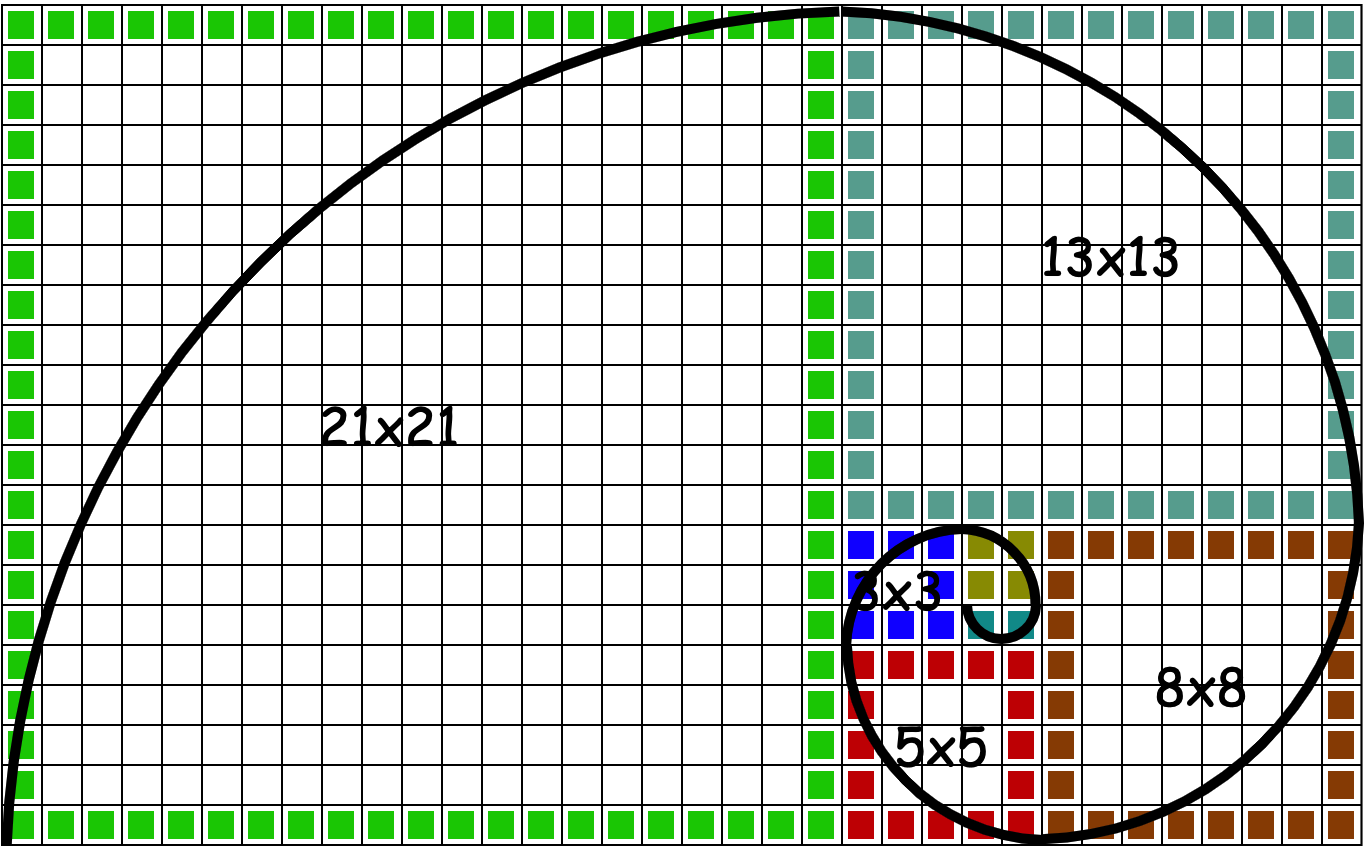F0 = 0，F1 = 1，Fn = Fn-1 + Fn-2

F0 F1 F2 F3 F4 F5 F6 F7 F8 F9 F10 F11 F12 F13 F14 F15 F16 F17 F18 F19
0 1 1 2 3 5 8 13 21 34 55 89 144 233 377 610 987 1597 2584 4181
• 从 F2 开始任意一位都是前两位之和。
• 从 F2 开始任意一位与前一位相比的比值，都无限趋近于 (√5 - 1)/2 = 0.618 因此基于黄金分割的计算应用，也被称为斐波那契应用。

## # 二、斐波那契计算

### # 1. 循环计算

``````public double fibonacci(int n) {
double currentVal = 1;
double previousVal = 0;
if (n == 1) return 1;
int iterationsCounter = n - 1;
while (iterationsCounter > 0) {
currentVal += previousVal;
previousVal = currentVal - previousVal;
iterationsCounter -= 1;
}
return currentVal;
}
``````
1
2
3
4
5
6
7
8
9
10
11
12

### # 2. 递归计算

``````public int fibonacciRecursion(int n) {
if (n == 1 || n == 2) {
return 1;
} else {
return (fibonacciRecursion(n - 1) + fibonacciRecursion(n - 2));
}
}
``````
1
2
3
4
5
6
7

### # 3. 比奈公式

``````public double fibonacciClosedForm(long position) {
int maxPosition = 75;
if (position < 1 || position > maxPosition) {
throw new RuntimeException("Can't handle position smaller than 1 or greater than 75");
}
double sqrt = Math.sqrt(5);
double phi = (1 + sqrt) / 2;
return Math.floor((Math.pow(phi, position)) / sqrt + 0.5);
}
``````
1
2
3
4
5
6
7
8
9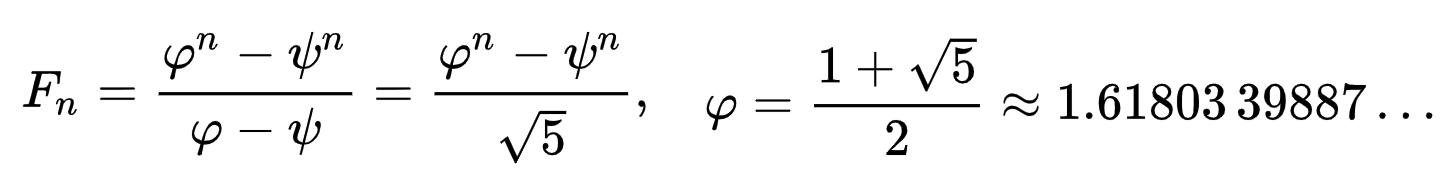• 封闭式表达式：与由具有常数系数的线性递归定义的每个序列一样，斐波那契数具有封闭形式的表达式。它被称为比奈公式，以法国数学家雅克·菲利普·玛丽·比内命名，尽管亚伯拉罕·德·莫弗和丹尼尔·伯努利已经知道它。

## # 三、散列函数分类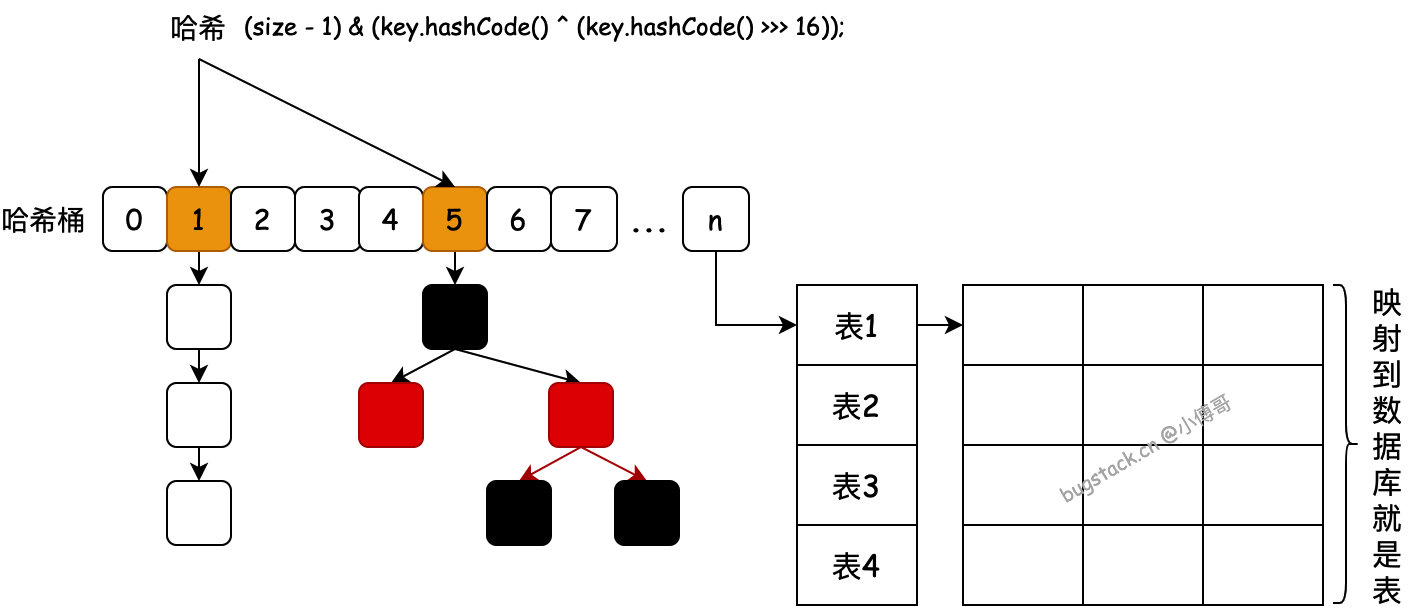### # 2. 乘法散列

• 用关键字k乘上常数`A(0<A<1)`，并去除kA的小数部分
• 用m乘以这个值，再取结果的底`floor` 公式： `h(K)=Math.floor[m(aK mod 1)]`

• 假设某计算机的字长为 ww 位，而 kk 正好可容于一个字中`(k<2wk<2w)`
• 现在选取范围`[0，2w]`内的任意数值 ss，k×sk×s 即可用`R1·2w+R0R1·2w+R0`来表示
• 因此`(k·A)mod1=k·s/2w(k·A)mod1=k·s/2w`就是将`k×sk×s`整体向右平移 ww 位，此时R0R0即为小数部分
• 再乘以 2m2m 相当于左移 mm 位，散列值`h(k)h(k)` 为 R0R0 的前 m 位。

## # 四、雪崩标准测试

SAC 建立在完整性和雪崩的概念之上，由 Webster 和 Tavares 于 1985 年引入。SAC 的高阶概括涉及多个输入位。满足最高阶 SAC 的最大非线性函数，也称为“完全非线性”函数。

1. 准备10万个单词用作样本数据。
2. 对比测试除法散列、乘法散列、斐波那契散列。
3. 基于条件1、2，对数据通过不同的散列算法分两次路由到8库32表和16库32表中，验证每个区间内数据的变化数量，是否在50%左右。
4. 准备一个 excel 表，来做数据的统计计算。

``````public Map<Integer, Map<Integer, Integer>> hashFunction(int dbCount, int tbCount, Long hashIncrementVal, int hashType) {
int size = dbCount * tbCount;
System.out.print("库数：" + dbCount + " 表数：" + tbCount + " 总值：" + size + " 幂值：" + Math.log(size) / Math.log(2));

int HASH_INCREMENT = (int) ((null == hashIncrementVal ? size : hashIncrementVal) * (Math.sqrt(5) - 1) / 2);
System.out.print(" 黄金分割：" + HASH_INCREMENT + "/" + size + " = " + (double) HASH_INCREMENT / size);

Map<Integer, Map<Integer, Integer>> map = new ConcurrentHashMap<>();
System.out.println(" 单词总数：" + words.size() + "\r\n");

for (String word : words) {
int idx = 0;
switch (hashType) {
// 散列：斐波那契散列  int idx = (size - 1) & (word.hashCode() * HASH_INCREMENT + HASH_INCREMENT);
case 0:
idx = (word.hashCode() * HASH_INCREMENT) & (size - 1);
break;
// 散列：哈希散列 + 扰动函数
case 1:
idx = (size - 1) & (word.hashCode() ^ (word.hashCode() >>> 16));
break;
// 散列：哈希散列
case 2:
idx = (size - 1) & (word.hashCode()/* ^ (word.hashCode() >>> 16)*/);
break;
// 散列：整数求模
case 3:
idx = Math.abs(word.hashCode()) % size;
break;
}

// 计算路由索引
int dbIdx = idx / tbCount + 1;
int tbIdx = idx - tbCount * (dbIdx - 1);

// 保存路由结果
if (map.containsKey(dbIdx)) {
Map<Integer, Integer> dbCountMap = map.get(dbIdx);
if (dbCountMap.containsKey(tbIdx)) {
dbCountMap.put(tbIdx, dbCountMap.get(tbIdx) + 1);
} else {
dbCountMap.put(tbIdx, 1);
}
} else {
Map<Integer, Integer> dbCountMap = new HashMap<>();
dbCountMap.put(tbIdx, 1);
map.put(dbIdx, dbCountMap);
}
}
return map;
}
``````
1
2
3
4
5
6
7
8
9
10
11
12
13
14
15
16
17
18
19
20
21
22
23
24
25
26
27
28
29
30
31
32
33
34
35
36
37
38
39
40
41
42
43
44
45
46
47
48
49
50
51
52
• 整个方法的目的在于得出不同的哈希算法，对10万个单词散列到指定的分库分表中，所体现的结果。

### # 1. 斐波那契散列

#### # 1.1 最小黄金分割

``````@Test
public void test_hashFunction_0_hash_null() {
Map<Integer, Map<Integer, Integer>> map = fibonacci.hashFunction(8, 32, null, 0);
Set<Integer> keys = map.keySet();
for (Integer key : keys) {
Collection<Integer> values = map.get(key).values();
for (Integer v : values) {
System.out.print(v + " ");
}
System.out.println();
}
}
``````
1
2
3
4
5
6
7
8
9
10
11
12
``````库数：8 表数：32 总值：256 幂值：8.0 黄金分割：2147483647/256 = 8388607.99609375 单词总数：103976
``````
1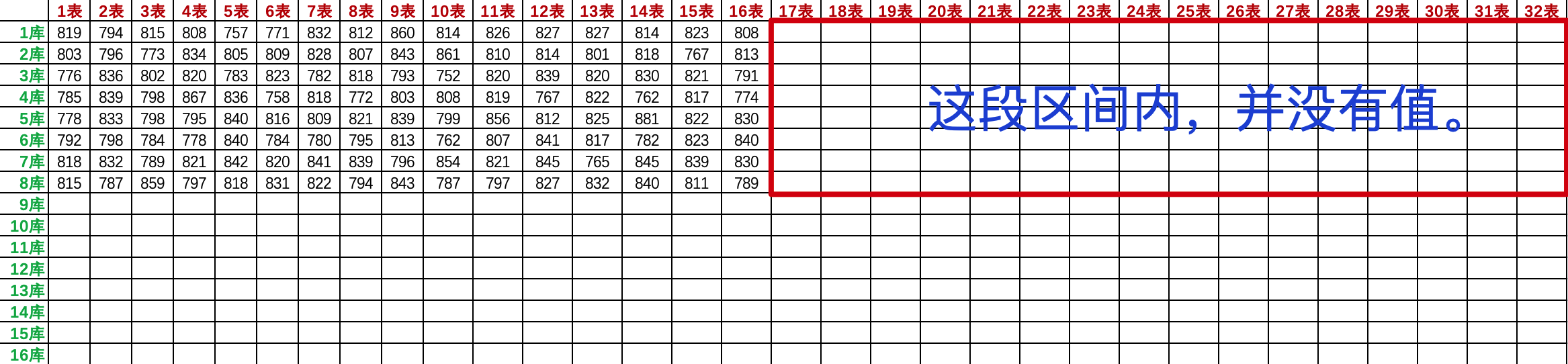#### # 1.2 最大黄金分割

``````@Test
public void test_hashFunction_0() {
Map<Integer, Map<Integer, Integer>> map = fibonacci.hashFunction(8, 32, 1L << 32, 0);
Set<Integer> keys = map.keySet();
for (Integer key : keys) {
Collection<Integer> values = map.get(key).values();
for (Integer v : values) {
System.out.print(v + " ");
}
System.out.println();
}
}
``````
1
2
3
4
5
6
7
8
9
10
11
12
• 分别测试 dbCount = 8、dbCount = 16
``````库数：8 表数：32 总值：512 幂值：9.0 黄金分割：2147483647/512 = 4194303.998046875 单词总数：103976

``````
1
2
3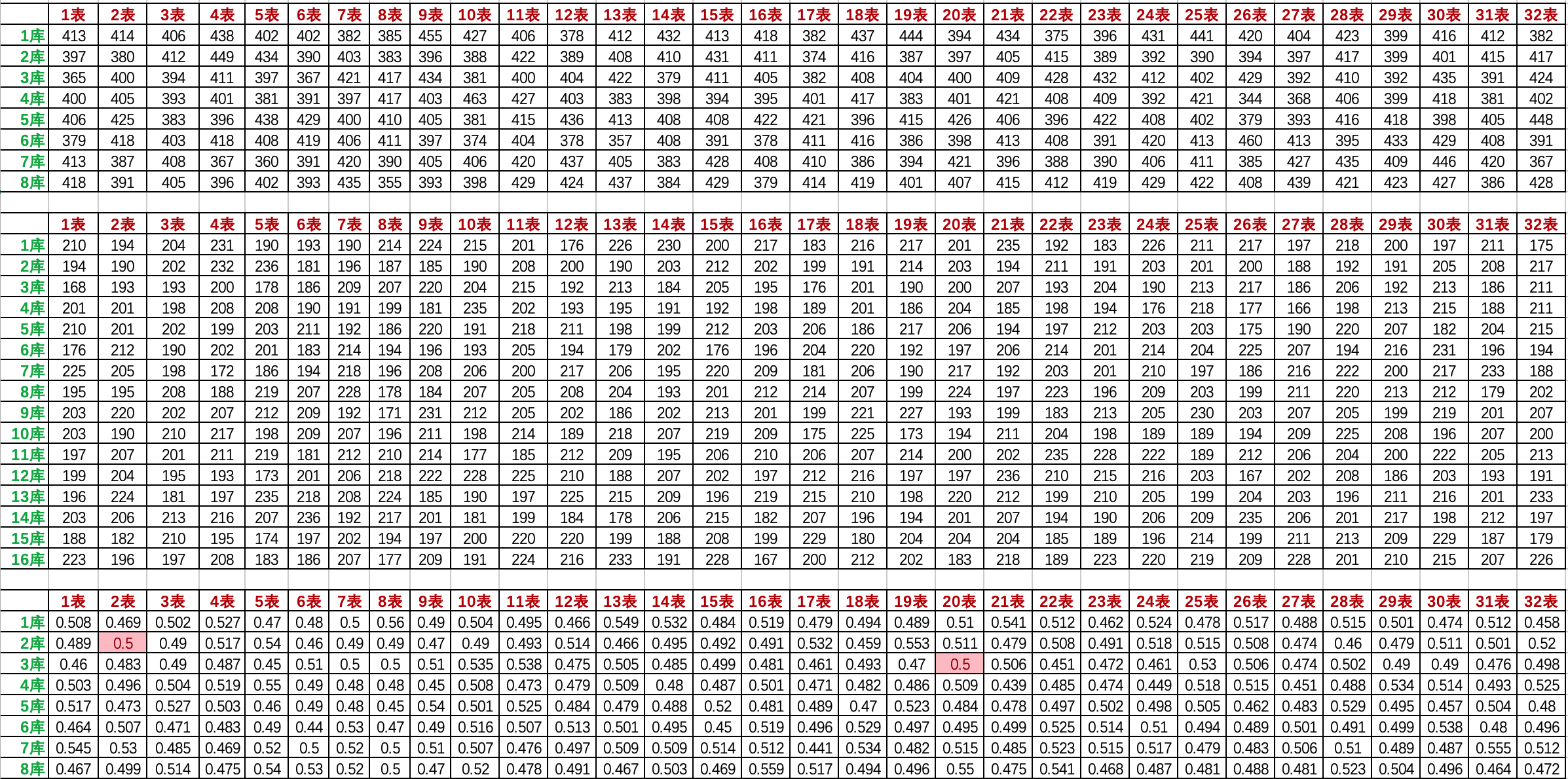• 从8库扩到16库以后，满足50%数据变化的，只有2库2表和3库20表。其他数据变化都不满足严格的雪崩测试。

#### # 1.3 任意扩容库表

``````    Map<Integer, Map<Integer, Integer>> map = fibonacci.hashFunction(9, 32, 1L << 32, 0);
Set<Integer> keys = map.keySet();
for (Integer key : keys) {
Collection<Integer> values = map.get(key).values();
for (Integer v : values) {
System.out.print(v + " ");
}
System.out.println();
}
}
``````
1
2
3
4
5
6
7
8
9
10
``````库数：9 表数：32 总值：512 幂值：9.0 黄金分割：2147483647/512 = 4194303.998046875 单词总数：103976
``````
1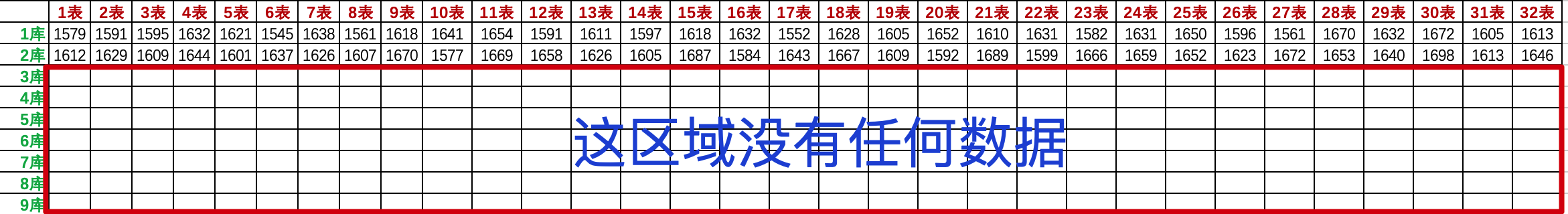• 因为9库不满足2的次幂，也就没法直接乘法散列。所以相当于斐波那契散列失效了。这如果是线上的生产环境，将发生灾难性的事故。

### # 2. 整数求模散列

#### # 2.1 基础散列计算

``````@Test
public void test_hashFunction_3() {
Map<Integer, Map<Integer, Integer>> map = fibonacci.hashFunction(8, 32, null, 3);
Set<Integer> keys = map.keySet();
for (Integer key : keys) {
Collection<Integer> values = map.get(key).values();
for (Integer v : values) {
System.out.print(v + " ");
}
System.out.println();
}
}
``````
1
2
3
4
5
6
7
8
9
10
11
12
• 分别测试 dbCount = 8、dbCount = 16
``````库数：8 表数：32 总值：512 幂值：9.0 黄金分割：2147483647/512 = 4194303.998046875 单词总数：103976

``````
1
2
3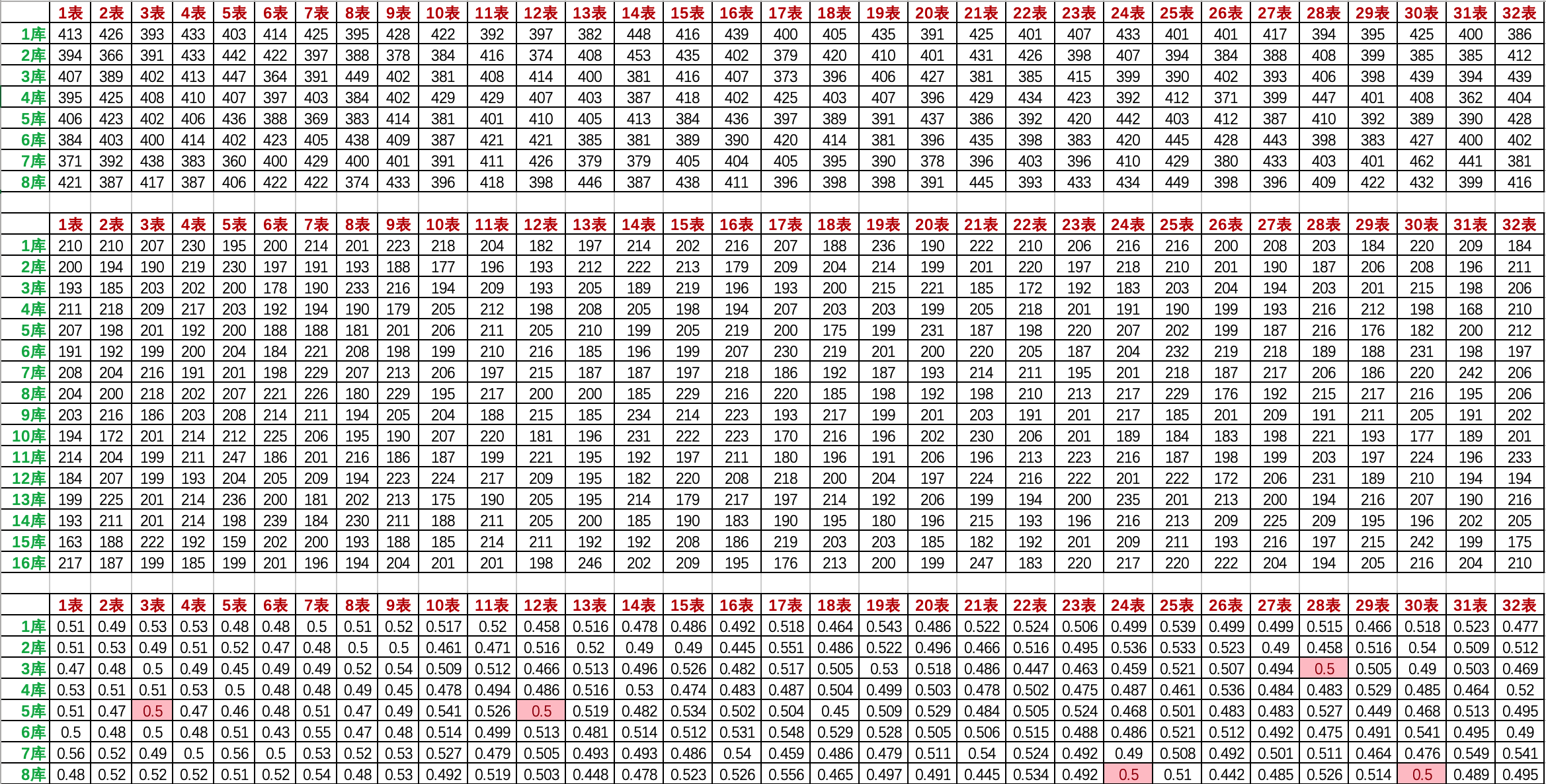• 在使用除法散列方式后，满足50%数据变化的有5个表。看着并不多，但这相当于是斐波那契散列下的3倍。同时其他表数据接近50%的也要大于斐波那契散列。

#### # 2.2 任意扩容计算

``````@Test
public void test_hashFunction_3() {
Map<Integer, Map<Integer, Integer>> map = fibonacci.hashFunction(9, 32, null, 3);
Set<Integer> keys = map.keySet();
for (Integer key : keys) {
Collection<Integer> values = map.get(key).values();
for (Integer v : values) {
System.out.print(v + " ");
}
System.out.println();
}
}
``````
1
2
3
4
5
6
7
8
9
10
11
12
``````库数：9 表数：32 总值：512 幂值：9.0 黄金分割：2147483647/512 = 4194303.998046875 单词总数：103976
``````
1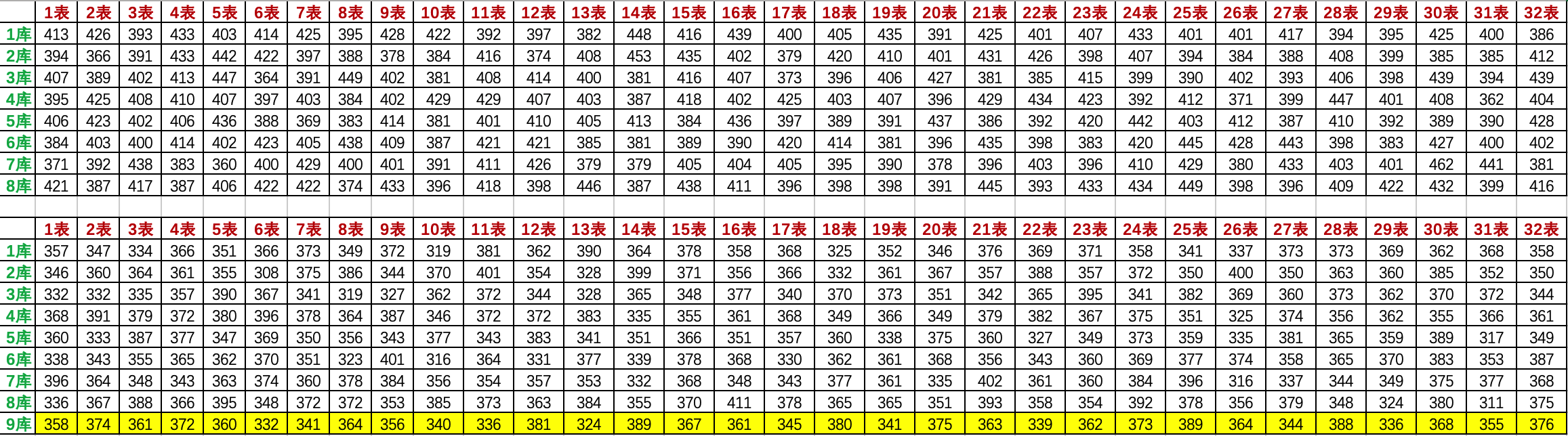• 103976 / (9 * 32) ≈ 361，那么也就说扩容后的数据，基本在361范围波动，就满足了均匀散列的目的。所以在数据库散列算法中，除法散列是较靠谱且稳定的。

## # 五、常见面试题

• 散列算法有哪些种？
• 你有了解过 `0x61c88647` 是怎么计算的吗？